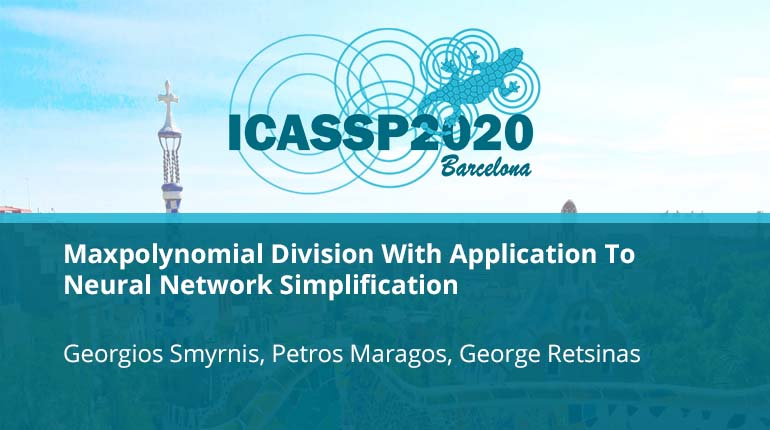# Maxpolynomial Division With Application To Neural Network Simplification

Collection:In this work, we further the link between neural networks with piecewise linear activations and tropical algebra. To that end, we introduce the process of Maxpolynomial Division, a geometric method which simulates division of polynomials in the max-plus s
• IEEE MemberUS \$11.00
• Society MemberUS \$0.00
• IEEE Student MemberUS \$11.00
• Non-IEEE MemberUS \$15.00
Purchase

## Maxpolynomial Division With Application To Neural Network Simplification

In this work, we further the link between neural networks with piecewise linear activations and tropical algebra. To that end, we introduce the process of Maxpolynomial Division, a geometric method which simulates division of polynomials in the max-plus s